Lec 19 - Subspaces and Spanning in a Vector Space

Wednesday, February 15, 2012

9:27 AMSubspaces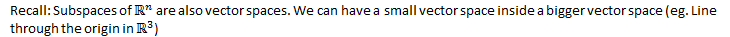This also happens in other vector spaces.

Definition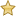Theorem: Subspace Test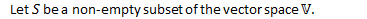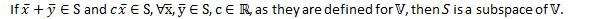Eg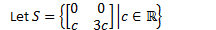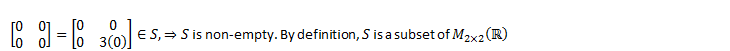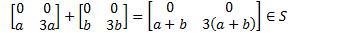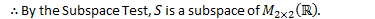Eg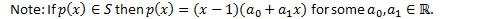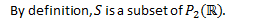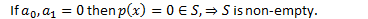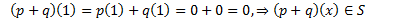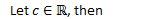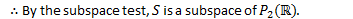Eg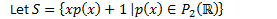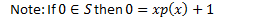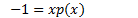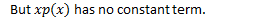Spanning

Definition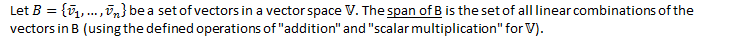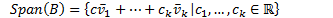Definition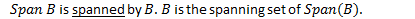Eg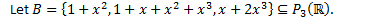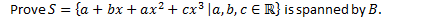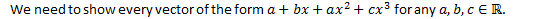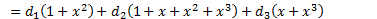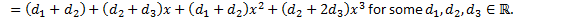We get the following system of linear equations: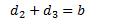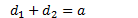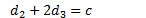Put in a matrix: (Drop the 3rd equation)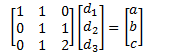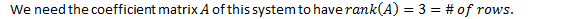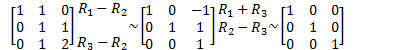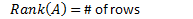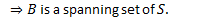Eg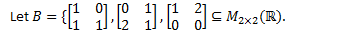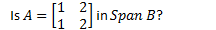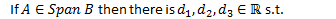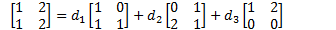We get the following system of linear equations: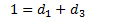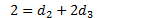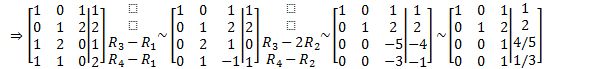The system is inconsistent.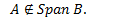Theorem:Proof: (by the subspace test)

Created by Tim Pei with Microsoft OneNote 2010
One place for all your notes and information